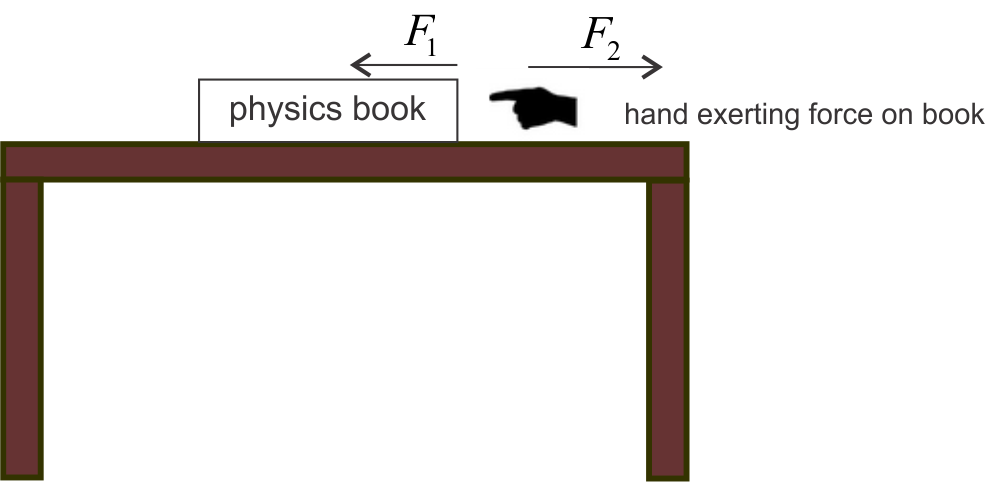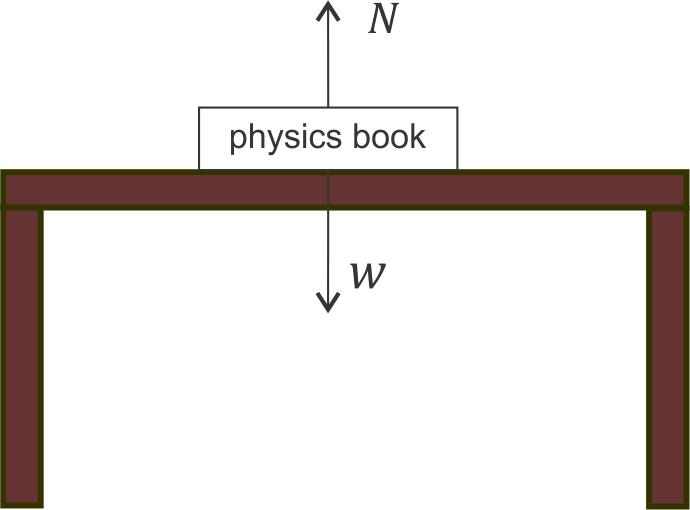Home » General » Newton’s Third Law of motion (action-reaction and examples)

# Newton’s Third Law of motion (action-reaction and examples)

## Newton’s Third Law of motion

Here in this article I presume that you have a introduction of Newtons first law of motion and Newton’s second law of motion.  Now Newtons first law and second law of motion deals with the effect of forces when they acts on a body. First law is about the change in the velocity of the body on the application of unbalanced forces and second law is gives the exact amount of the force needed to produce a given acceleration.

Here in this article we shall discuss about the Newton’s Third Law of motion which is the relation between the forces themselves. You must have observed that when a body exerts a force on another body , other body also exerts force on the first body. So here both he bodies are interacting with each other . Now Newton’s third law of motion states that

In any interaction between two bodies , force applied by the first body on the second is equal and opposite to the force applied by the second body on the first

This means that forces always occur in pairs. Some of the examples of Newton’s third law of motion are

1. Consider a book being placed on a table as shown below in the figureNow you push the book towards th eleft hand side by using your hand to apply force. According to Newton’s Law , the book also exerts force on your hand and the magnitude of the force applied by book on your hand is equal to the magnitude of force applied on the book by your hand.
So, $F_{1}=F_{2}$
where $F_{1}$ is the force applied by the hand on the book and $F_{2}$ is the force applied by the book on the hand.
2. When a man jumps from a boat to the shore, the boat moves away from him. The force he exerts on the boat (action) is responsible for its motion and his motion to the shore is due to the force of reaction exerted by the boat on him.
3. If you hit the wall with your fist, the wall also hits your fist with the same force , which you feel.

## Action and Reaction

To understand this consider two objects A and B. Whenever object A apply a force on object B , there is also a force applied by object B on object A. These two forces have equal magnitude and have opposite direction. Such a pair of forces , exerted by two bodies on each other , is called an action-reaction pair. Any one of the forces in the pair can be called action force and the other force would be called as reaction force.  Now we can state the Newton’s third law of motion in terms of action and reaction

Action and reaction are always equal and opposite.

Important thing to note about action -reaction forces is that they are two forces acting on two different bodies.

Here I would like to mention one more fact that is any pair of equal and opposite force is not an action reaction pair. For identifying action reaction pair you would have to identify the two interacting objects and deciding who is pushing on whom and in what direction. for further detail consider a book placed on the table as shown below in the figure.From this figure we can see that when a book is placed on the table two equal and opposite forces acts on it. First one is force $W$ that is acting along downward direction and is applied by the earth and second one is $N$ the normal force applied by the table in the upward direction. Now since the book is at rest on the table we can conclude that these two forces are equal in magnitude and opposite in direction. But these two forces are not action reaction pair as these forces are not applied by two bodies on each other. Here the force $N$ is applied by the  table on the book , the reaction to this force would be force applied by the book on the table. Similar is the case with the weight $W$ which is the force applied by the earth on the book and reaction to this force would be force applied by the book on the earth. so here you see that $N$ and $W$ are two equal and opposite forces but they do not form action reaction pair.

One more important thing to note about action reaction pair is that the acceleration produced by these forces on interacting objects is not same an the reason behind it is that there might be difference between masses of two interacting objects.

### Other recommended articles

For more notes on laws of motion you can consider visiting these links
[standout-css3-button href=”https://physicscatalyst.com/Class9/laws_of_motion.php”]Force class 9 notes[/standout-css3-button] [standout-css3-button href=”https://physicscatalyst.com/mech/force.php”]Force class 11/IITJEE notes[/standout-css3-button]

Subscribe
Notify of

This site uses Akismet to reduce spam. Learn how your comment data is processed.

1 Comment
Inline Feedbacks